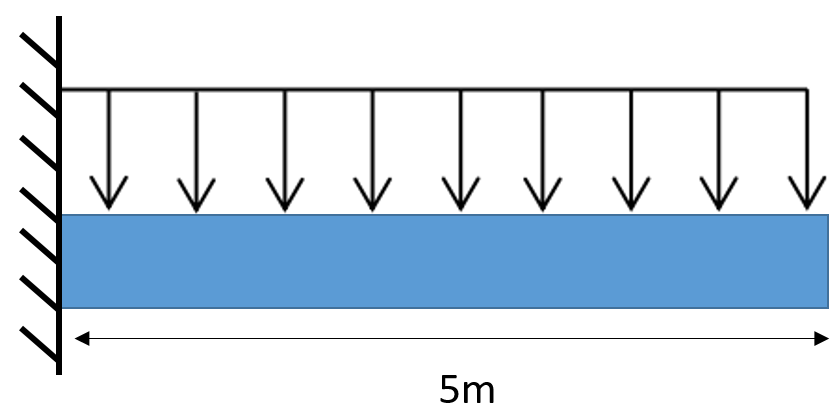## Cantilevered Beam

Calculate the deflection at the cantilevered beam's tip if a 7,000 N/m distributed load is applied. Assume E*I = 6.4x10^6 N*m^2.Hint
$$\upsilon_{max}=\frac{-wL^{4}}{8EI}$$$where $$w$$ is the distributed load, $$L$$ is the beam’s length, $$E$$ is the modulus of elasticity, and $$I$$ is the moment of inertia. Hint 2 The final answer should be negative to indicate a downward deflection. Referencing the cantilevered beam slopes and deflections section in the FE handbook (or any other ref table), the deflection formula is: $$\upsilon_{max}=\frac{-wL^{4}}{8EI}$$$
where $$w$$ is the distributed load, $$L$$ is the beam’s length, $$E$$ is the modulus of elasticity, and $$I$$ is the moment of inertia.
$$\upsilon_{max}=\frac{-7,000N/m\cdot (5m)^{4}}{8\cdot 6.4\times 10^6N\cdot m^2}$$$$$\upsilon_{max}=-\frac{4,375,000}{51,200,000}\:m=-0.085\:m$$$
(Note: the negative sign (-) indicates the deflection is downwards.)
-0.085 m